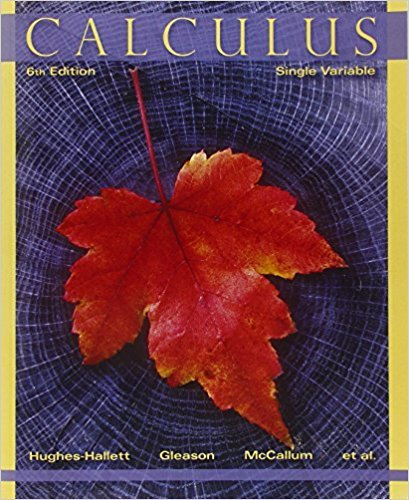×
×

# Solutions for Chapter 7.4: ALGEBRAIC IDENTITIES AND TRIGONOMETRIC SUBSTITUTIONS## Full solutions for Calculus: Single Variable | 6th Edition

ISBN: 9780470888643Solutions for Chapter 7.4: ALGEBRAIC IDENTITIES AND TRIGONOMETRIC SUBSTITUTIONS

Solutions for Chapter 7.4
4 5 0 390 Reviews
29
0
##### ISBN: 9780470888643

Calculus: Single Variable was written by and is associated to the ISBN: 9780470888643. This expansive textbook survival guide covers the following chapters and their solutions. Since 90 problems in chapter 7.4: ALGEBRAIC IDENTITIES AND TRIGONOMETRIC SUBSTITUTIONS have been answered, more than 35266 students have viewed full step-by-step solutions from this chapter. Chapter 7.4: ALGEBRAIC IDENTITIES AND TRIGONOMETRIC SUBSTITUTIONS includes 90 full step-by-step solutions. This textbook survival guide was created for the textbook: Calculus: Single Variable , edition: 6.

Key Calculus Terms and definitions covered in this textbook
• Absolute maximum

A value ƒ(c) is an absolute maximum value of ƒ if ƒ(c) ? ƒ(x) for all x in the domain of ƒ.

• Ambiguous case

The case in which two sides and a nonincluded angle can determine two different triangles

• Damping factor

The factor Ae-a in an equation such as y = Ae-at cos bt

• End behavior

The behavior of a graph of a function as.

• Explicitly defined sequence

A sequence in which the kth term is given as a function of k.

• Independent variable

Variable representing the domain value of a function (usually x).

• Infinite discontinuity at x = a

limx:a + x a ƒ(x) = q6 or limx:a - ƒ(x) = q.

• Jump discontinuity at x a

limx:a - ƒ1x2 and limx:a + ƒ1x2 exist but are not equal

• Linear regression equation

Equation of a linear regression line

• Lower bound test for real zeros

A test for finding a lower bound for the real zeros of a polynomial

• Multiplication principle of probability

If A and B are independent events, then P(A and B) = P(A) # P(B). If Adepends on B, then P(A and B) = P(A|B) # P(B)

• NDER ƒ(a)

See Numerical derivative of ƒ at x = a.

• Nonsingular matrix

A square matrix with nonzero determinant

• Parameter interval

See Parametric equations.

• Partial fraction decomposition

See Partial fractions.

• Placebo

In an experimental study, an inactive treatment that is equivalent to the active treatment in every respect except for the factor about which an inference is to be made. Subjects in a blind experiment do not know if they have been given the active treatment or the placebo.

• Positive numbers

Real numbers shown to the right of the origin on a number line.

• Singular matrix

A square matrix with zero determinant

• Transpose of a matrix

The matrix AT obtained by interchanging the rows and columns of A.

• Wrapping function

The function that associates points on the unit circle with points on the real number line

×